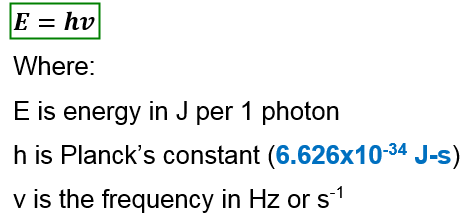# Problem: An x-ray has a wavelength of 1.3 Å. Calculate the energy (in J) of one photon of this radiation.

###### FREE Expert Solution

The energy of the radiation can be calculated using the equation:88% (3 ratings)###### Problem Details

An x-ray has a wavelength of 1.3 Å. Calculate the energy (in J) of one photon of this radiation.